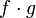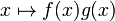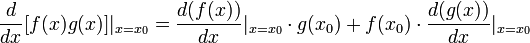# Product rule for differentiation

(diff) ← Older revision | Latest revision (diff) | Newer revision → (diff)

## Statement for two functions

### Verbal statement

If two (possibly equal) functions are differentiable at a given real number, then their pointwise product is also differentiable at that number and the derivative of the product is the sum of two terms: the derivative of the first function times the second function and the first function times the derivative of the second function.

### Statement with symbols

Suppose$f$ and$g$ are functions, both of which are differentiable at a real number$x = x_0$. Then, the product function$f \cdot g$, defined as$x \mapsto f(x)g(x)$ is also differentiable at$x$, and the derivative at$x_0$ is given as follows:$\! \frac{d}{dx} [f(x)g(x)]|_{x = x_0} = f'(x_0)g(x_0) + f(x_0)g'(x_0)$

or equivalently:$\! \frac{d}{dx} [f(x)g(x)]|_{x = x_0} = \frac{d(f(x))}{dx}|_{x=x_0} \cdot g(x_0) + f(x_0)\cdot \frac{d(g(x))}{dx}|_{x = x_0}$

If we consider the general expressions rather than evaluation at a particular point$x_0$, we can rewrite the above as:$\! \frac{d}{dx}[f(x)g(x)] = f'(x)g(x) + f(x)g'(x)$

or equivalently:$(f \cdot g)' = (f' \cdot g) + (f \cdot g')$